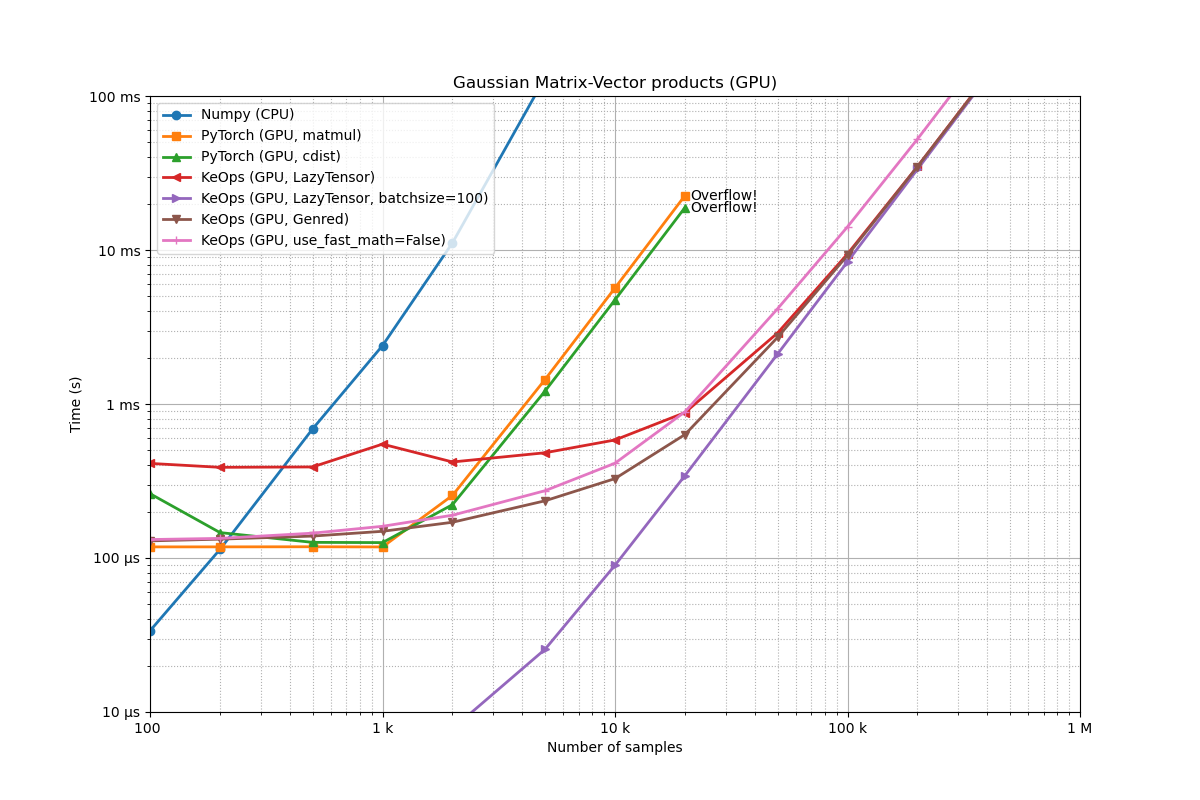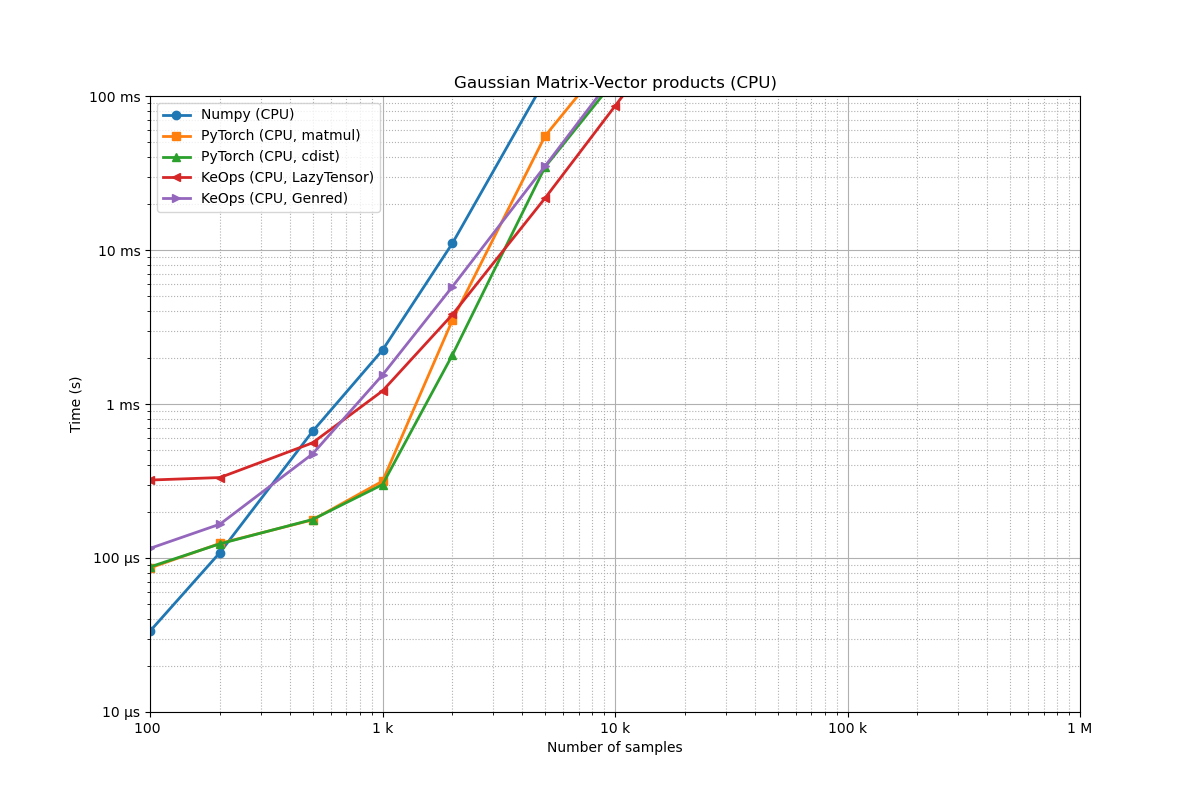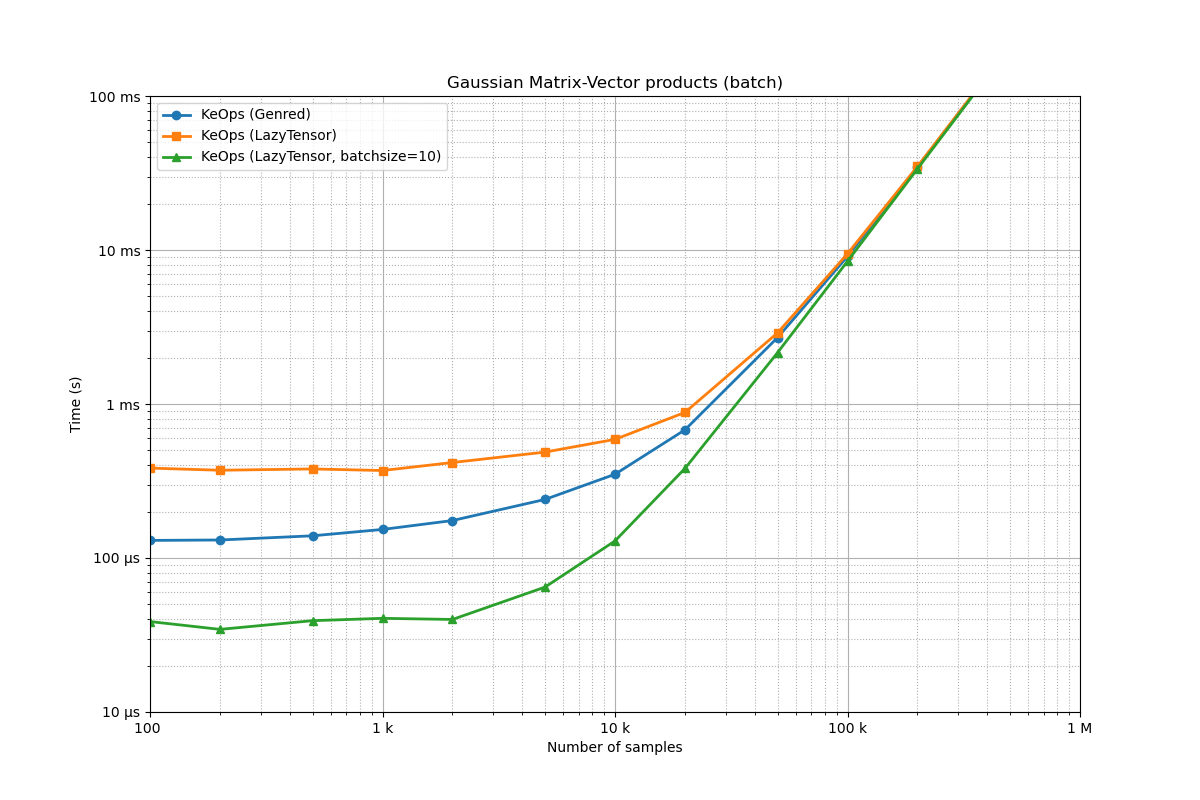# Scaling up Gaussian convolutions on 3D point clouds

Let’s compare the performances of PyTorch and KeOps on simple Gaussian RBF kernel products, as the number of samples grows from 100 to 1,000,000.

Note

In this demo, we use exact bruteforce computations (tensorized for PyTorch and online for KeOps), without leveraging any multiscale or low-rank (Nystroem/multipole) decomposition of the Kernel matrix. We are working on providing transport support for these approximations in KeOps.

## Setup

import numpy as np
import torch
from matplotlib import pyplot as plt

from benchmark_utils import flatten, random_normal, full_benchmark

use_cuda = torch.cuda.is_available()


Benchmark specifications:

# Numbers of samples that we'll loop upon:
problem_sizes = flatten(
[[1 * 10**k, 2 * 10**k, 5 * 10**k] for k in [2, 3, 4, 5]] + [[10**6]]
)
D = 3  # We work with 3D points


Synthetic dataset. Feel free to use a Stanford Bunny, or whatever!

def generate_samples(N, device="cuda", lang="torch", batchsize=1, **kwargs):
"""Generates two point clouds x, y and a scalar signal b of size N.

Args:
N (int): number of point.
device (str, optional): "cuda", "cpu", etc. Defaults to "cuda".
lang (str, optional): "torch", "numpy", etc. Defaults to "torch".
batchsize (int, optional): number of experiments to run in parallel. Defaults to None.

Returns:
3-uple of arrays: x, y, b
"""
randn = random_normal(device=device, lang=lang)

x = randn((batchsize, N, D))
y = randn((batchsize, N, D))
b = randn((batchsize, N, 1))

return x, y, b


Define a simple Gaussian RBF product, using a tensorized implementation. Note that expanding the squared norm $$\|x-y\|^2$$ as a sum $$\|x\|^2 - 2 \langle x, y \rangle + \|y\|^2$$ allows us to leverage the fast matrix-matrix product of the BLAS/cuBLAS libraries.

def gaussianconv_numpy(x, y, b, **kwargs):
"""(1,N,D), (1,N,D), (1,N,1) -> (1,N,1)"""

# N.B.: NumPy does not really support batch matrix multiplications:
x, y, b = x.squeeze(0), y.squeeze(0), b.squeeze(0)

D_xx = np.sum((x**2), axis=-1)[:, None]  # (N,1)
D_xy = x @ y.T  # (N,D) @ (D,M) = (N,M)
D_yy = np.sum((y**2), axis=-1)[None, :]  # (1,M)
D_xy = D_xx - 2 * D_xy + D_yy  # (N,M)
K_xy = np.exp(-D_xy)  # (B,N,M)

return K_xy @ b

def gaussianconv_pytorch(x, y, b, tf32=False, **kwargs):
"""(B,N,D), (B,N,D), (B,N,1) -> (B,N,1)"""

# If False, we stick to float32 computations.
# If True, we use TensorFloat32 whenever possible.
torch.backends.cuda.matmul.allow_tf32 = tf32

D_xx = (x * x).sum(-1).unsqueeze(2)  # (B,N,1)
D_xy = torch.matmul(x, y.permute(0, 2, 1))  # (B,N,D) @ (B,D,M) = (B,N,M)
D_yy = (y * y).sum(-1).unsqueeze(1)  # (B,1,M)
D_xy = D_xx - 2 * D_xy + D_yy  # (B,N,M)
K_xy = (-D_xy).exp()  # (B,N,M)

return K_xy @ b  # (B,N,1)


Define a simple Gaussian RBF product, using an online implementation:

from pykeops.torch import generic_sum

fun_gaussianconv_keops = generic_sum(
"Exp(-SqDist(X,Y)) * B",  # Formula
"A = Vi(1)",  # Output
"X = Vi({})".format(D),  # 1st argument
"Y = Vj({})".format(D),  # 2nd argument
"B = Vj(1)",  # 3rd argument
)

def gaussianconv_keops(x, y, b, backend="GPU", **kwargs):
"""(B,N,D), (B,N,D), (B,N,1) -> (B,N,1)"""
x, y, b = x.squeeze(), y.squeeze(), b.squeeze()
return fun_gaussianconv_keops(x, y, b, backend=backend)


Finally, perform the same operation with our high-level pykeops.torch.LazyTensor wrapper:

from pykeops.torch import LazyTensor

def gaussianconv_lazytensor(x, y, b, backend="GPU", **kwargs):
"""(B,N,D), (B,N,D), (B,N,1) -> (B,N,1)"""
x_i = LazyTensor(x.unsqueeze(-2))  # (B, M, 1, D)
y_j = LazyTensor(y.unsqueeze(-3))  # (B, 1, N, D)
D_ij = ((x_i - y_j) ** 2).sum(-1)  # (B, M, N, 1)
K_ij = (-D_ij).exp()  # (B, M, N, 1)
S_ij = K_ij * b.unsqueeze(-3)  # (B, M, N, 1) * (B, 1, N, 1)
return S_ij.sum(dim=2, backend=backend)


## NumPy vs. PyTorch vs. KeOps (Gpu)

if use_cuda:
routines = [
(gaussianconv_numpy, "Numpy (CPU)", {"lang": "numpy"}),
(gaussianconv_pytorch, "PyTorch (GPU, TF32=False)", {"tf32": False}),
(gaussianconv_pytorch, "PyTorch (GPU, TF32=True)", {"tf32": True}),
(gaussianconv_keops, "KeOps (GPU)", {}),
]

full_benchmark(
"Gaussian Matrix-Vector products (GPU)",
routines,
generate_samples,
problem_sizes=problem_sizes,
max_time=1,
)Benchmarking : Gaussian Matrix-Vector products (GPU) ===============================
Numpy (CPU) -------------
1x100 loops of size  100 :   1x100x  44.9 µs
1x100 loops of size  200 :   1x100x 185.4 µs
1x100 loops of size  500 :   1x100x 908.5 µs
1x100 loops of size   1 k:   1x100x   3.4 ms
1x100 loops of size   2 k:   1x100x  16.0 ms
1x 10 loops of size   5 k:   1x 10x 148.1 ms
1x  1 loops of size  10 k:   1x  1x 417.3 ms
1x  1 loops of size  20 k:   1x  1x    1.7 s
** Too slow!
PyTorch (GPU, TF32=False) -------------
1x100 loops of size  100 :   1x100x 117.1 µs
1x100 loops of size  200 :   1x100x 117.2 µs
1x100 loops of size  500 :   1x100x 115.8 µs
1x100 loops of size   1 k:   1x100x 116.2 µs
1x100 loops of size   2 k:   1x100x 151.7 µs
1x100 loops of size   5 k:   1x100x 806.3 µs
1x100 loops of size  10 k:   1x100x   2.9 ms
1x100 loops of size  20 k:   1x100x  11.4 ms
1x 10 loops of size  50 k:   1x 10x  71.8 ms
CUDA out of memory. Tried to allocate 37.25 GiB (GPU 0; 79.15 GiB total capacity; 74.56 GiB already allocated; 1.35 GiB free; 74.58 GiB reserved in total by PyTorch) If reserved memory is >> allocated memory try setting max_split_size_mb to avoid fragmentation.  See documentation for Memory Management and PYTORCH_CUDA_ALLOC_CONF
** Runtime error!
PyTorch (GPU, TF32=True) -------------
1x100 loops of size  100 :   1x100x 118.2 µs
1x100 loops of size  200 :   1x100x 120.8 µs
1x100 loops of size  500 :   1x100x 114.4 µs
1x100 loops of size   1 k:   1x100x 117.3 µs
1x100 loops of size   2 k:   1x100x 130.4 µs
1x100 loops of size   5 k:   1x100x 791.3 µs
1x100 loops of size  10 k:   1x100x   2.9 ms
1x100 loops of size  20 k:   1x100x  11.3 ms
1x 10 loops of size  50 k:   1x 10x  71.8 ms
CUDA out of memory. Tried to allocate 37.25 GiB (GPU 0; 79.15 GiB total capacity; 74.56 GiB already allocated; 1.35 GiB free; 74.58 GiB reserved in total by PyTorch) If reserved memory is >> allocated memory try setting max_split_size_mb to avoid fragmentation.  See documentation for Memory Management and PYTORCH_CUDA_ALLOC_CONF
** Runtime error!
KeOps (GPU) -------------
1x100 loops of size  100 :   1x100x 107.3 µs
1x100 loops of size  200 :   1x100x 111.1 µs
1x100 loops of size  500 :   1x100x 125.1 µs
1x100 loops of size   1 k:   1x100x 142.1 µs
1x100 loops of size   2 k:   1x100x 175.3 µs
1x100 loops of size   5 k:   1x100x 283.2 µs
1x100 loops of size  10 k:   1x100x 460.3 µs
1x100 loops of size  20 k:   1x100x 816.7 µs
1x100 loops of size  50 k:   1x100x   3.7 ms
1x100 loops of size 100 k:   1x100x  11.5 ms
1x 10 loops of size 200 k:   1x 10x  43.5 ms
1x 10 loops of size 500 k:   1x 10x 263.1 ms
1x  1 loops of size   1 M:   1x  1x    1.0 s
** Too slow!


## NumPy vs. PyTorch vs. KeOps (Cpu)

routines = [
(gaussianconv_numpy, "Numpy (CPU)", {"device": "cpu", "lang": "numpy"}),
(gaussianconv_pytorch, "PyTorch (CPU)", {"device": "cpu"}),
(gaussianconv_keops, "KeOps (CPU)", {"device": "cpu", "backend": "CPU"}),
]

full_benchmark(
"Gaussian Matrix-Vector products (CPU)",
routines,
generate_samples,
problem_sizes=problem_sizes,
max_time=1,
)Benchmarking : Gaussian Matrix-Vector products (CPU) ===============================
Numpy (CPU) -------------
1x100 loops of size  100 :   1x100x  43.4 µs
1x100 loops of size  200 :   1x100x 183.2 µs
1x100 loops of size  500 :   1x100x 889.8 µs
1x100 loops of size   1 k:   1x100x   3.4 ms
1x100 loops of size   2 k:   1x100x  15.6 ms
1x 10 loops of size   5 k:   1x 10x 146.5 ms
1x  1 loops of size  10 k:   1x  1x 416.1 ms
1x  1 loops of size  20 k:   1x  1x    1.7 s
** Too slow!
PyTorch (CPU) -------------
1x100 loops of size  100 :   1x100x  93.1 µs
1x100 loops of size  200 :   1x100x 134.7 µs
1x100 loops of size  500 :   1x100x 316.6 µs
1x100 loops of size   1 k:   1x100x 709.6 µs
1x100 loops of size   2 k:   1x100x   5.2 ms
1x100 loops of size   5 k:   1x100x  69.9 ms
1x 10 loops of size  10 k:   1x 10x 278.9 ms
1x  1 loops of size  20 k:   1x  1x    1.1 s
** Too slow!
KeOps (CPU) -------------
1x100 loops of size  100 :   1x100x 109.6 µs
1x100 loops of size  200 :   1x100x 169.7 µs
1x100 loops of size  500 :   1x100x 621.1 µs
1x100 loops of size   1 k:   1x100x   2.2 ms
1x100 loops of size   2 k:   1x100x   8.6 ms
1x100 loops of size   5 k:   1x100x  53.8 ms
1x 10 loops of size  10 k:   1x 10x 214.0 ms
1x  1 loops of size  20 k:   1x  1x 855.4 ms
1x  1 loops of size  50 k:   1x  1x    5.3 s
** Too slow!


## Genred vs. LazyTensor vs. batched LazyTensor

if use_cuda:
routines = [
(gaussianconv_keops, "KeOps (Genred)", {}),
(gaussianconv_lazytensor, "KeOps (LazyTensor)", {}),
(
gaussianconv_lazytensor,
"KeOps (LazyTensor, batchsize=10)",
{"batchsize": 10},
),
]

full_benchmark(
"Gaussian Matrix-Vector products (batch)",
routines,
generate_samples,
problem_sizes=problem_sizes,
max_time=1,
)

plt.show()Benchmarking : Gaussian Matrix-Vector products (batch) ===============================
KeOps (Genred) -------------
1x100 loops of size  100 :   1x100x 111.4 µs
1x100 loops of size  200 :   1x100x 115.7 µs
1x100 loops of size  500 :   1x100x 129.8 µs
1x100 loops of size   1 k:   1x100x 150.9 µs
1x100 loops of size   2 k:   1x100x 191.7 µs
1x100 loops of size   5 k:   1x100x 322.1 µs
1x100 loops of size  10 k:   1x100x 539.6 µs
1x100 loops of size  20 k:   1x100x 912.3 µs
1x100 loops of size  50 k:   1x100x   3.8 ms
1x100 loops of size 100 k:   1x100x  11.5 ms
1x 10 loops of size 200 k:   1x 10x  43.4 ms
1x 10 loops of size 500 k:   1x 10x 262.5 ms
1x  1 loops of size   1 M:   1x  1x    1.0 s
** Too slow!
KeOps (LazyTensor) -------------
1x100 loops of size  100 :   1x100x 313.0 µs
1x100 loops of size  200 :   1x100x 314.7 µs
1x100 loops of size  500 :   1x100x 327.7 µs
1x100 loops of size   1 k:   1x100x 348.4 µs
1x100 loops of size   2 k:   1x100x 384.7 µs
1x100 loops of size   5 k:   1x100x 495.3 µs
1x100 loops of size  10 k:   1x100x 678.0 µs
1x100 loops of size  20 k:   1x100x   1.0 ms
1x100 loops of size  50 k:   1x100x   4.0 ms
1x100 loops of size 100 k:   1x100x  12.0 ms
1x 10 loops of size 200 k:   1x 10x  44.8 ms
1x 10 loops of size 500 k:   1x 10x 269.4 ms
1x  1 loops of size   1 M:   1x  1x    1.0 s
** Too slow!
KeOps (LazyTensor, batchsize=10) -------------
10x100 loops of size  100 :  10x100x  31.1 µs
10x100 loops of size  200 :  10x100x  31.7 µs
10x100 loops of size  500 :  10x100x  32.7 µs
10x100 loops of size   1 k:  10x100x  34.6 µs
10x100 loops of size   2 k:  10x100x  41.8 µs
10x100 loops of size   5 k:  10x100x  69.1 µs
10x100 loops of size  10 k:  10x100x 149.7 µs
10x100 loops of size  20 k:  10x100x 476.6 µs
10x100 loops of size  50 k:  10x100x   2.7 ms
10x100 loops of size 100 k:  10x100x  10.5 ms
10x 10 loops of size 200 k:  10x 10x  41.3 ms
10x 10 loops of size 500 k:  10x 10x 255.8 ms
10x  1 loops of size   1 M:  10x  1x    1.0 s
** Too slow!


Total running time of the script: ( 2 minutes 26.659 seconds)

Gallery generated by Sphinx-Gallery